14 Fluid Mechanics

14.4 Archimedes’ Principle and Buoyancy

Learning Objectives

By the end of this section, you will be able to:

• Define buoyant force
• State Archimedes’ principle
• Describe the relationship between density and Archimedes’ principle

When placed in a fluid, some objects float due to a buoyant force. Where does this buoyant force come from? Why is it that some things float and others do not? Do objects that sink get any support at all from the fluid? Is your body buoyed by the atmosphere, or are only helium balloons affected ((Figure))?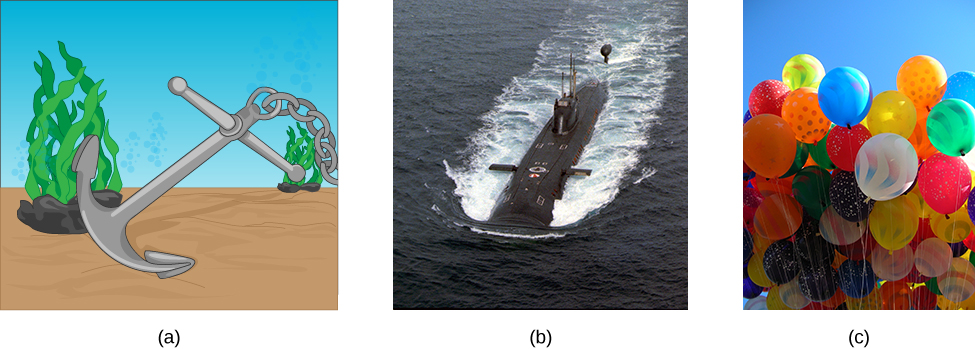Figure 14.19 (a) Even objects that sink, like this anchor, are partly supported by water when submerged. (b) Submarines have adjustable density (ballast tanks) so that they may float or sink as desired. (c) Helium-filled balloons tug upward on their strings, demonstrating air’s buoyant effect. (credit b: modification of work by Allied Navy; credit c: modification of work by “Crystl”/Flickr)

Answers to all these questions, and many others, are based on the fact that pressure increases with depth in a fluid. This means that the upward force on the bottom of an object in a fluid is greater than the downward force on top of the object. There is an upward force, or buoyant force, on any object in any fluid ((Figure)). If the buoyant force is greater than the object’s weight, the object rises to the surface and floats. If the buoyant force is less than the object’s weight, the object sinks. If the buoyant force equals the object’s weight, the object can remain suspended at its present depth. The buoyant force is always present, whether the object floats, sinks, or is suspended in a fluid.

Buoyant Force

The buoyant force is the upward force on any object in any fluid.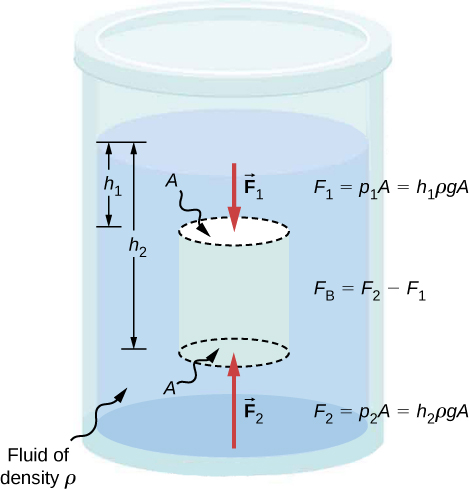Figure 14.20 Pressure due to the weight of a fluid increases with depth because     . This change in pressure and associated upward force on the bottom of the cylinder are greater than the downward force on the top of the cylinder. The differences in the force results in the buoyant force     . (Horizontal forces cancel.)

Archimedes’ Principle

Just how large a force is buoyant force? To answer this question, think about what happens when a submerged object is removed from a fluid, as in (Figure). If the object were not in the fluid, the space the object occupied would be filled by fluid having a weight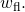This weight is supported by the surrounding fluid, so the buoyant force must equal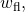the weight of the fluid displaced by the object.

Archimedes’ Principle

The buoyant force on an object equals the weight of the fluid it displaces. In equation form, Archimedes’ principle is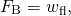where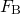is the buoyant force and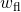is the weight of the fluid displaced by the object.

This principle is named after the Greek mathematician and inventor Archimedes (ca. 287–212 BCE), who stated this principle long before concepts of force were well established.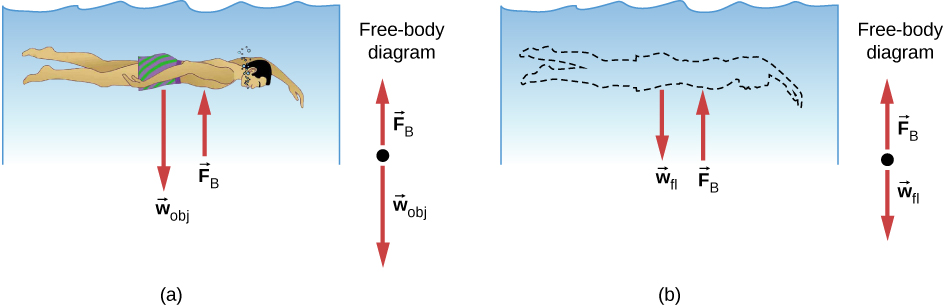Figure 14.21 (a) An object submerged in a fluid experiences a buoyant force     If     is greater than the weight of the object, the object rises. If     is less than the weight of the object, the object sinks. (b) If the object is removed, it is replaced by fluid having weight     Since this weight is supported by surrounding fluid, the buoyant force must equal the weight of the fluid displaced.

Archimedes’ principle refers to the force of buoyancy that results when a body is submerged in a fluid, whether partially or wholly. The force that provides the pressure of a fluid acts on a body perpendicular to the surface of the body. In other words, the force due to the pressure at the bottom is pointed up, while at the top, the force due to the pressure is pointed down; the forces due to the pressures at the sides are pointing into the body.

Since the bottom of the body is at a greater depth than the top of the body, the pressure at the lower part of the body is higher than the pressure at the upper part, as shown in (Figure). Therefore a net upward force acts on the body. This upward force is the force of buoyancy, or simply buoyancy.

The exclamation “Eureka” (meaning “I found it”) has often been credited to Archimedes as he made the discovery that would lead to Archimedes’ principle. Some say it all started in a bathtub. To read the story, visit NASA or explore Scientific American to learn more.

Density and Archimedes’ Principle

If you drop a lump of clay in water, it will sink. But if you mold the same lump of clay into the shape of a boat, it will float. Because of its shape, the clay boat displaces more water than the lump and experiences a greater buoyant force, even though its mass is the same. The same is true of steel ships.

The average density of an object is what ultimately determines whether it floats. If an object’s average density is less than that of the surrounding fluid, it will float. The reason is that the fluid, having a higher density, contains more mass and hence more weight in the same volume. The buoyant force, which equals the weight of the fluid displaced, is thus greater than the weight of the object. Likewise, an object denser than the fluid will sink.

The extent to which a floating object is submerged depends on how the object’s density compares to the density of the fluid. In (Figure), for example, the unloaded ship has a lower density and less of it is submerged compared with the same ship when loaded. We can derive a quantitative expression for the fraction submerged by considering density. The fraction submerged is the ratio of the volume submerged to the volume of the object, or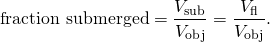The volume submerged equals the volume of fluid displaced, which we call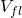. Now we can obtain the relationship between the densities by substituting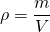into the expression. This gives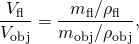where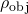is the average density of the object and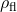is the density of the fluid. Since the object floats, its mass and that of the displaced fluid are equal, so they cancel from the equation, leaving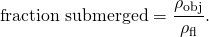We can use this relationship to measure densities.

Example

Calculating Average Density

Suppose a 60.0-kg woman floats in fresh water with 97.0% of her volume submerged when her lungs are full of air. What is her average density?

Strategy

We can find the woman’s density by solving the equation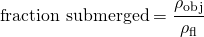for the density of the object. This yields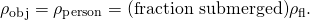We know both the fraction submerged and the density of water, so we can calculate the woman’s density.

Solution

Entering the known values into the expression for her density, we obtain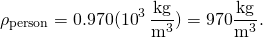Significance

The woman’s density is less than the fluid density. We expect this because she floats.

Numerous lower-density objects or substances float in higher-density fluids: oil on water, a hot-air balloon in the atmosphere, a bit of cork in wine, an iceberg in salt water, and hot wax in a “lava lamp,” to name a few. A less obvious example is mountain ranges floating on the higher-density crust and mantle beneath them. Even seemingly solid Earth has fluid characteristics.

Measuring Density

One of the most common techniques for determining density is shown in (Figure).Figure 14.23 (a) A coin is weighed in air. (b) The apparent weight of the coin is determined while it is completely submerged in a fluid of known density. These two measurements are used to calculate the density of the coin.

An object, here a coin, is weighed in air and then weighed again while submerged in a liquid. The density of the coin, an indication of its authenticity, can be calculated if the fluid density is known. We can use this same technique to determine the density of the fluid if the density of the coin is known.

All of these calculations are based on Archimedes’ principle, which states that the buoyant force on the object equals the weight of the fluid displaced. This, in turn, means that the object appears to weigh less when submerged; we call this measurement the object’s apparent weight. The object suffers an apparent weight loss equal to the weight of the fluid displaced. Alternatively, on balances that measure mass, the object suffers an apparent mass loss equal to the mass of fluid displaced. That is, apparent weight loss equals weight of fluid displaced, or apparent mass loss equals mass of fluid displaced.

Summary

• Buoyant force is the net upward force on any object in any fluid. If the buoyant force is greater than the object’s weight, the object will rise to the surface and float. If the buoyant force is less than the object’s weight, the object will sink. If the buoyant force equals the object’s weight, the object can remain suspended at its present depth. The buoyant force is always present and acting on any object immersed either partially or entirely in a fluid.
• Archimedes’ principle states that the buoyant force on an object equals the weight of the fluid it displaces.

Conceptual Questions

More force is required to pull the plug in a full bathtub than when it is empty. Does this contradict Archimedes’ principle? Explain your answer.

Not at all. Pascal’s principle says that the change in the pressure is exerted through the fluid. The reason that the full tub requires more force to pull the plug is because of the weight of the water above the plug.

Do fluids exert buoyant forces in a “weightless” environment, such as in the space shuttle? Explain your answer.

Will the same ship float higher in salt water than in freshwater? Explain your answer.

The buoyant force is equal to the weight of the fluid displaced. The greater the density of the fluid, the less fluid that is needed to be displaced to have the weight of the object be supported and to float. Since the density of salt water is higher than that of fresh water, less salt water will be displaced, and the ship will float higher.

Marbles dropped into a partially filled bathtub sink to the bottom. Part of their weight is supported by buoyant force, yet the downward force on the bottom of the tub increases by exactly the weight of the marbles. Explain why.

Problems

What fraction of ice is submerged when it floats in freshwater, given the density of water at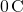is very close to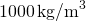?

If a person’s body has a density of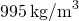, what fraction of the body will be submerged when floating gently in (a) freshwater? (b) In salt water with a density of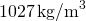?

a. 99.5% submerged; b. 96.9% submerged

A rock with a mass of 540 g in air is found to have an apparent mass of 342 g when submerged in water. (a) What mass of water is displaced? (b) What is the volume of the rock? (c) What is its average density? Is this consistent with the value for granite?

Archimedes’ principle can be used to calculate the density of a fluid as well as that of a solid. Suppose a chunk of iron with a mass of 390.0 g in air is found to have an apparent mass of 350.5 g when completely submerged in an unknown liquid. (a) What mass of fluid does the iron displace? (b) What is the volume of iron, using its density as given in (Figure)? (c) Calculate the fluid’s density and identify it.

a. 39.5 g; b.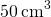; c.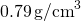; ethyl alcohol

Calculate the buoyant force on a 2.00-L helium balloon. (b) Given the mass of the rubber in the balloon is 1.50 g, what is the net vertical force on the balloon if it is let go? Neglect the volume of the rubber.

What is the density of a woman who floats in fresh water with

*** QuickLaTeX cannot compile formula:
$4.00\text{%}$

*** Error message:
File ended while scanning use of \text@.
Emergency stop.

of her volume above the surface? (This could be measured by placing her in a tank with marks on the side to measure how much water she displaces when floating and when held under water.) (b) What percent of her volume is above the surface when she floats in seawater?

a.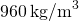; b. 6.34%; She floats higher in seawater.

A man has a mass of 80 kg and a density of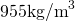(excluding the air in his lungs). (a) Calculate his volume. (b) Find the buoyant force air exerts on him. (c) What is the ratio of the buoyant force to his weight?

A simple compass can be made by placing a small bar magnet on a cork floating in water. (a) What fraction of a plain cork will be submerged when floating in water? (b) If the cork has a mass of 10.0 g and a 20.0-g magnet is placed on it, what fraction of the cork will be submerged? (c) Will the bar magnet and cork float in ethyl alcohol?

a. 0.24; b. 0.68; c. Yes, the cork will float in ethyl alcohol.

What percentage of an iron anchor’s weight will be supported by buoyant force when submerged in salt water?

Referring to (Figure), prove that the buoyant force on the cylinder is equal to the weight of the fluid displaced (Archimedes’ principle). You may assume that the buoyant force is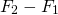and that the ends of the cylinder have equal areas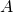. Note that the volume of the cylinder (and that of the fluid it displaces) equals.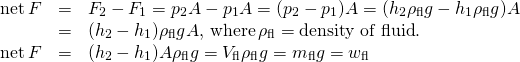A 75.0-kg man floats in freshwater with 3.00% of his volume above water when his lungs are empty, and 5.00% of his volume above water when his lungs are full. Calculate the volume of air he inhales—called his lung capacity—in liters. (b) Does this lung volume seem reasonable?

Glossary

Archimedes’ principle
buoyant force on an object equals the weight of the fluid it displaces
buoyant force
net upward force on any object in any fluid due to the pressure difference at different depths